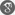##### Cite as:
Pankaj Chettri, Subodh Gurung, Khusboo Katwal, ps-ro Fuzzy Open(Closed) Functions and ps-ro Fuzzy Semi-Homeomorphism, Int. J. Anal. Appl., 9 (2) (2015), 121-128.

#### Abstract

The aim of this paper is to introduce and characterize some new class of functions in a fuzzy topological space termed as ps-ro fuzzy open(closed) functions, ps-ro fuzzy pre semiopen functions and ps-ro fuzzy semi-homeomorphism. The interrelation among these concepts and also their relations with the parallel existing concepts are established. It is also shown with the help of examples that these newly introduced concepts are independent of the well known existing allied concepts.

#### References

1. Chang, C. L., Fuzzy topological spaces, J. Math. Anal. Appl.,24(1968), 182-190.2. Chettri, P., Gurung, S. and Halder, S., On ps-ro Semiopen Fuzzy Set and ps-ro Fuzzy Semicontinuous, Semiopen functions, Tbilisi Mathematical Journal., 7(1)(2014) 87-97.3. Chettri, P., Katwal, K. and Gurung, S., On ps-ro fuzzy irresolute functions, Pre Print.4. Deb Ray, A. and Chettri, P., On pseudo δ-open fuzzy sets and pseudo fuzzy δ-continuous functions, International Journal of Contemporary Mathematical Sciences., 5(29)(2010), 1403- 1411.5. Deb Ray, A and Chettri, P., Fuzzy pseudo nearly compact spaces and ps-ro continuous functions, The Journal of Fuzzy Mathematics., 19(3) (2011), 737-746.6. Deb Ray, A. and Chettri, P., Further on fuzzy pseudo near compactness and ps-ro fuzzy continuous functions, Pre Print.7. Kohli, J. K. and Prasannan, A. R., Starplus-Compactness and Starplus-Compact Open Fuzzy Topologies on Function Spaces, J. Math. Anal. Appl., 254(2001), 87-100.8. Lowen, R., Fuzzy Topological Spaces and Fuzzy Compactness, J. Math. Anal. Appl., 56(1976), 621-633.9. Malghan, S. R. and Benchalli, S.S., Open Maps, Closed Maps and Local Compactness in Fuzzy Topological Spaces, J. Math. Anal. Appl., 99(1984), 338-349.10. Yalvac,T.H., Semi-interior and Semi-closure of a Fuzzy Set, J. Math. Anal. Appl., 132(1988), 356-364.11. Zadeh, L. A., Fuzzy Sets, Information and Control., 8(1965), 338-353.